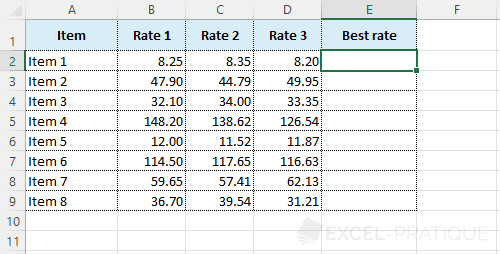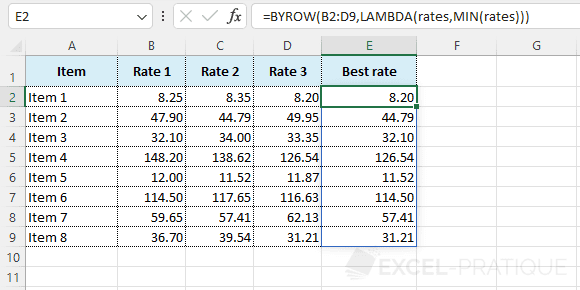# Excel Function: BYROW

The BYROW function applies a LAMBDA function to each row and returns an array of results.

Prerequisite: understand the LAMBDA function.

Usage:

`=BYROW(array, LAMBDA)`

## Usage example

The objective here is to list with a single formula the lowest rate for each item:Start by entering the BYROW function followed by the array that contains the different rates:

``=BYROW(B2:D9``

Then add the LAMBDA function and choose a variable name (for example "rates"):

``=BYROW(B2:D9,LAMBDA(rates``

And finally, add the MIN function to get the lowest rate:

``=BYROW(B2:D9,LAMBDA(rates,MIN(rates)))``

The BYROW function will then go through the entered array (B2:D9) row by row and return the smallest value among those of the current row: